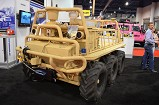OX5 by Lockheed Martin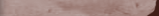• WHO IS MOAB JEEPER?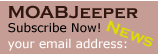Home
> Jeep Articles > General - Editorials > Winches In Hell - The Numbers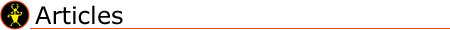# General Articles - Editorials

## Winches In Hell - The Numbers

Article written by MOABJEEPER Staff

##### Here it is...

Below you will find the testing numbers for the finishers.

 Amperage Chicago Electric Superwinch Warn 50' pull at 15° 207 score=1 242 score=3 219 score=2 155' pull at 24° and 18° 192 score=1 213 score=2 335 score=3 141' (Hells Gate) 270 score=1 281 score=2 307 score=3 35' at 55° 310 score=1 336 score=2 438 score=3 Total = 4 Ranked 1 Total = 9 Ranked 2 Total = 11 Ranked 3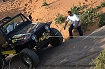Temperature after Pull Chicago Electric Superwinch Warn 50' pull at 15° 74.4 score=3 66.2 score=2 62.6 score=1 155' pull at 24° and 18° 105.3 score=2 111.3 score=3 98.2 score=1 141' (Hells Gate) 168.2 score=3 147.1 score=2 127.2 score=1 35' at 55° 90.1 score=1 92.6 score=2 105.8 score=3 Total = 9 Ranked 2 Total = 9 Ranked 2 Total = 6 Ranked 1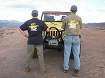Time of Pull (not line speed) Chicago Electric Superwinch Warn 50' pull at 15° 4:45 score=3 2:27 score=1 2:33 score=2 155' pull at 24° and 18° 13:55 score=3 6:35 score=2 6:32 score=1 141' (Hells Gate) 10:27 score=3 6:32 score=2 6:00 score=1 35' at 55° 3:06 score=3 2:01 score=2 1:56 score=1 Total = 12 Ranked 3 Total = 7 Ranked 2 Total = 5 Ranked 1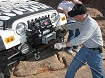Warranty Chicago Electric Superwinch Warn 90 Days 1 Year 1 Year Electrical Limited Lifetime Mechanical Ranked 3 Ranked 2 Ranked 1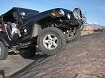Price per Pound Chicago Electric Superwinch Warn 599.99 ÷ 9000 =.066 611.98 ÷ 9000 =.067 1112.39 ÷ 9500 =.117 Ranked 1 Ranked 2 Ranked 3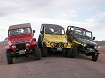Total Points per Category Chicago Electric Superwinch Warn Amperage Raw=4 Composite=1 Raw=9 Composite=2 Raw=11 Composite=3 Temp after Pull Raw=9 Composite=2 Raw=9 Composite=2 Raw=6 Composite=1 Pull Speed Raw=12 Composite=3 Raw=7 Composite=2 Raw=5 Composite=1 Warranty Raw=3 Composite=3 Raw=2 Composite=2 Raw=1 Composite=1 Price per Pound Raw=1 Composite=1 Raw=2 Composite=2 Raw=3 Composite=3 Total Score Raw=29 Composite=10 Raw=29 Composite=10 Raw=26 Composite=9 Rank by the #'s 2nd 2nd 1st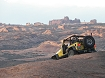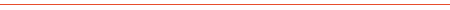#### Final Thoughts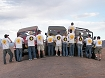####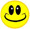Our Thank You's!

Thank you to everyone involved recording the data.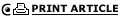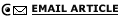ALL Editorials :

 © 2003-2008 Moab Jeeper Magazine. All rights reserved. Disclaimer and Privacy Policy apply to this site.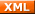Jeep®, Wrangler, Cherokee, and Grand Cherokee are copyrighted and trademarked to Daimler-Chrysler Corporation. MoabJeeper Magazine is not in any way associated with the Daimler-Chrysler Corp.